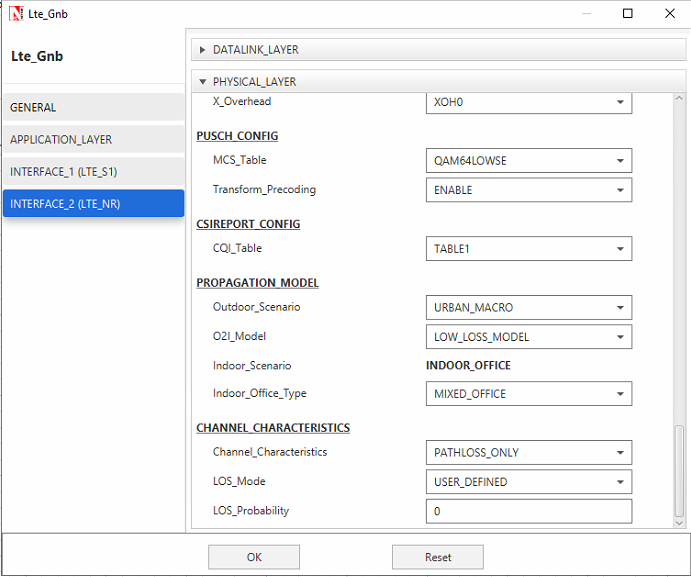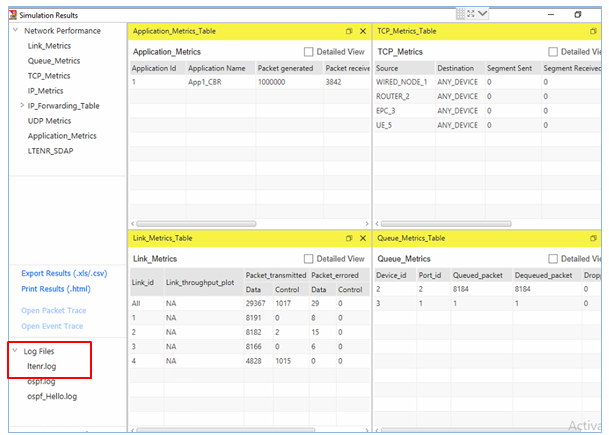The height of the gNB is a user defined value. When the chosen scenario is Urban Macro , the height of the gNB that a user would typically enter is  25m, which is the value provided in the standard.

However, NetSim doesn't strictly enforce this 25m value.

This means that a user can enter a different value. Based on the value entered NetSim would calculate the pathloss per  the following set of equations.When the input to the h_BS is entered as 40, it overwrites the default value of 25 (hence for the calculation of pathloss h_BS is taken as 40m)

The d3D parameter which is used to calculate the pathloss in LOS and NLOS depends on the hBS value.Therefore the values of pathloss depend on the user input hBS value.

If you wish to simulate the LOS and NLOS to check Propagation loss values for h_BS values of 25m and 40m, the steps below can be followed.

LOS
Under the gNB properties,
1.Enter the gNB height
2.Select channel characteristics as PATHLOSS_ONLY
3.Select LOS Mode as USER_DEFINED
4.Enter the LOS Probability as 1
5.Note down the pathloss value from the ltenr log fileNLOS
Under the gNB properties,
1.Enter the gNB height
2.Select channel characteristics as PATHLOSS_ONLY
3.Select LOS Mode as USER_DEFINED
4.Enter the LOS Probability as 0
5.Note down the pathloss value from the ltenr log fileCheck the propagation loss in the ltenr log file